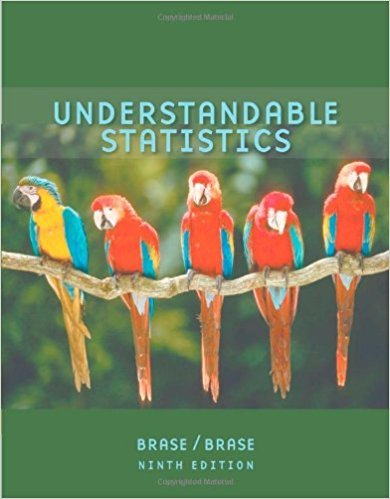×
×

# Solutions for Chapter 12.1: NONPARAMETRIC STATISTICS## Full solutions for Understandable Statistics | 9th Edition

ISBN: 9780618949922Solutions for Chapter 12.1: NONPARAMETRIC STATISTICS

Solutions for Chapter 12.1
4 5 0 397 Reviews
19
3
##### ISBN: 9780618949922

This textbook survival guide was created for the textbook: Understandable Statistics, edition: 9. This expansive textbook survival guide covers the following chapters and their solutions. Chapter 12.1: NONPARAMETRIC STATISTICS includes 12 full step-by-step solutions. Understandable Statistics was written by and is associated to the ISBN: 9780618949922. Since 12 problems in chapter 12.1: NONPARAMETRIC STATISTICS have been answered, more than 35307 students have viewed full step-by-step solutions from this chapter.

Key Statistics Terms and definitions covered in this textbook
• 2 k factorial experiment.

A full factorial experiment with k factors and all factors tested at only two levels (settings) each.

• Asymptotic relative eficiency (ARE)

Used to compare hypothesis tests. The ARE of one test relative to another is the limiting ratio of the sample sizes necessary to obtain identical error probabilities for the two procedures.

• Attribute control chart

Any control chart for a discrete random variable. See Variables control chart.

• Bernoulli trials

Sequences of independent trials with only two outcomes, generally called “success” and “failure,” in which the probability of success remains constant.

• Causal variable

When y fx = ( ) and y is considered to be caused by x, x is sometimes called a causal variable

• Central composite design (CCD)

A second-order response surface design in k variables consisting of a two-level factorial, 2k axial runs, and one or more center points. The two-level factorial portion of a CCD can be a fractional factorial design when k is large. The CCD is the most widely used design for itting a second-order model.

• Chance cause

The portion of the variability in a set of observations that is due to only random forces and which cannot be traced to speciic sources, such as operators, materials, or equipment. Also called a common cause.

• Combination.

A subset selected without replacement from a set used to determine the number of outcomes in events and sample spaces.

• Comparative experiment

An experiment in which the treatments (experimental conditions) that are to be studied are included in the experiment. The data from the experiment are used to evaluate the treatments.

• Conditional mean

The mean of the conditional probability distribution of a random variable.

• Continuous distribution

A probability distribution for a continuous random variable.

• Distribution function

Another name for a cumulative distribution function.

• Error mean square

The error sum of squares divided by its number of degrees of freedom.

• Estimator (or point estimator)

A procedure for producing an estimate of a parameter of interest. An estimator is usually a function of only sample data values, and when these data values are available, it results in an estimate of the parameter of interest.

• Expected value

The expected value of a random variable X is its long-term average or mean value. In the continuous case, the expected value of X is E X xf x dx ( ) = ?? ( ) ? ? where f ( ) x is the density function of the random variable X.

• F distribution.

The distribution of the random variable deined as the ratio of two independent chi-square random variables, each divided by its number of degrees of freedom.

• Fixed factor (or fixed effect).

In analysis of variance, a factor or effect is considered ixed if all the levels of interest for that factor are included in the experiment. Conclusions are then valid about this set of levels only, although when the factor is quantitative, it is customary to it a model to the data for interpolating between these levels.

• Forward selection

A method of variable selection in regression, where variables are inserted one at a time into the model until no other variables that contribute signiicantly to the model can be found.

• Geometric mean.

The geometric mean of a set of n positive data values is the nth root of the product of the data values; that is, g x i n i n = ( ) = / w 1 1 .

• Hat matrix.

In multiple regression, the matrix H XXX X = ( ) ? ? -1 . This a projection matrix that maps the vector of observed response values into a vector of itted values by yˆ = = X X X X y Hy ( ) ? ? ?1 .

×